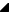﻿ TRSGPCanvas.Polygon(TPoint[]) Method# TRSGPCanvas.Polygon(TPoint[]) Method

Draws a series of lines on the canvas connecting the points passed in and closing the shape by drawing a line from the last point to the first point.

Implements drawing a closed, many-sided shape on the canvas, using the value of Pen. After drawing the complete shape, Polygon fills the shape using the current brush.

The Points parameter is an array of points that give the vertices of the polygon. The first point is always connected to the last point.

To draw a polygon on the canvas, without filling it, use the Polyline method, specifying the first point a second time, at the end.Note

In Delphi, you can use the Slice function to pass a portion of an array of points to the Polygon method. For example, to form a polygon using the first ten points from an array of 100 points, use the Slice function as follows: Canvas.Polygon(Slice(PointArray, 10));

Namespace: RSGdiPlusGraphicsSyntax

Parameters

Points

Type: array of TPointSee Also

RiverSoftAVG SVG Component Library (RSCL) © 2013-2016, Thomas G. Grubb